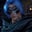Related Tags

# What is a max function in Haskell?Gutha Vamsi Krishna

## Overview

The max function is used to get the maximum of two arguments. We can get the maximum of two integers, two floats, or two characters.

### Syntax

max first_argument second_argument

### Parameters and return value

This function takes two parameter values to compare and returns the greater value.

Let's have a look at the example below.

### Example

main::IO()

main = do
-- get max of two integers
print(max 5 10)

-- get max of two floats
print(max 154.0 34.0)

-- get max of two characters
print(max 'a' 'A')

### Explanation

In the above code:

• Line 5: We use the max function to get the max of two integer values.
• Line 8: We use the max function to get the max of two float values.
• Line 11: We use the max function to get the max of two characters. We also use the ASCII value to compare the characters.

RELATED TAGS

CONTRIBUTORGutha Vamsi Krishna
RELATED COURSES

View all Courses

Keep Exploring

Learn in-demand tech skills in half the time